• Slides: 20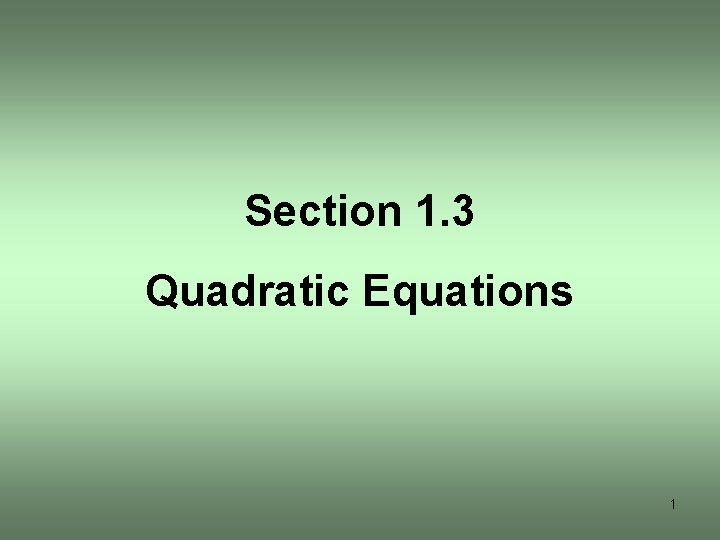Section 1. 3 Quadratic Equations 1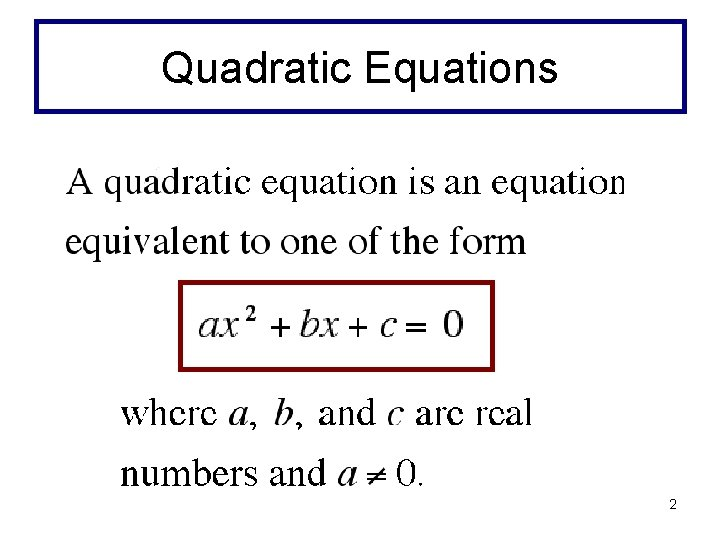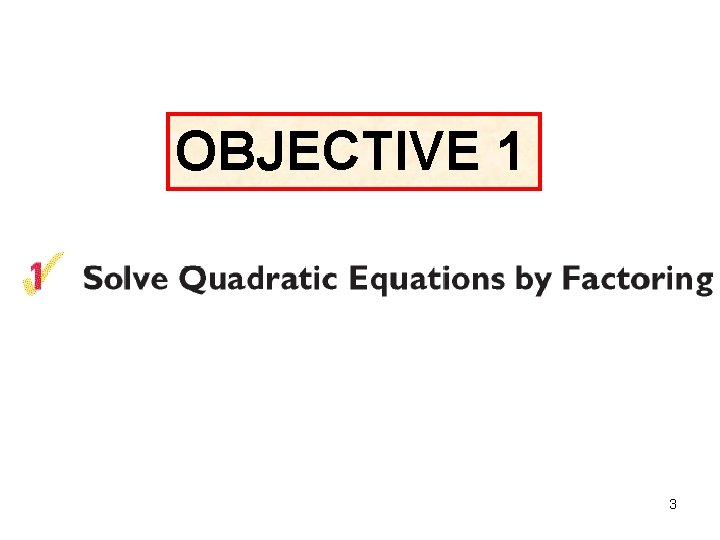OBJECTIVE 1 3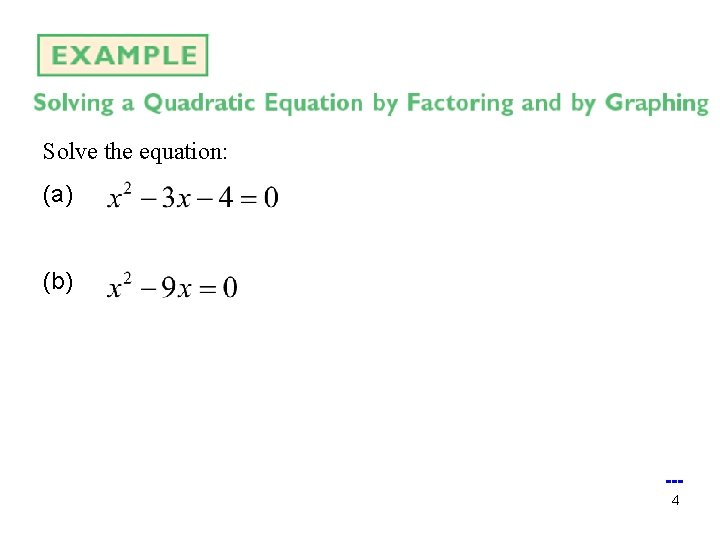Solve the equation: (a) (b) --4Solve the equation: --5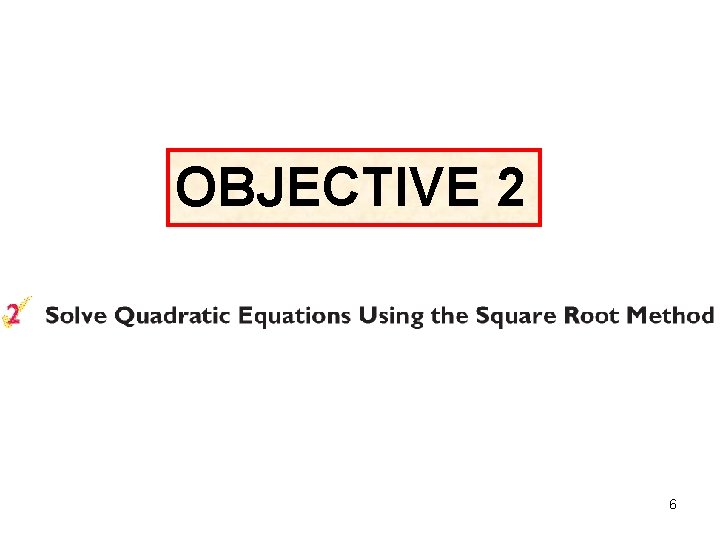OBJECTIVE 2 6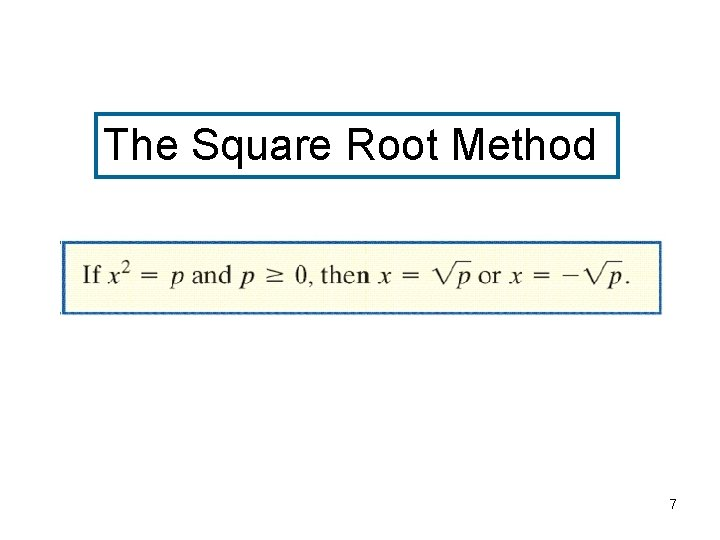The Square Root Method 7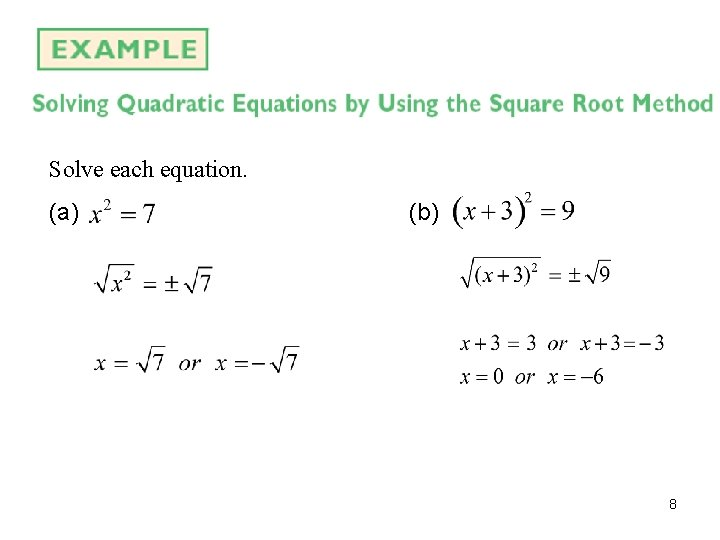Solve each equation. (a) (b) 8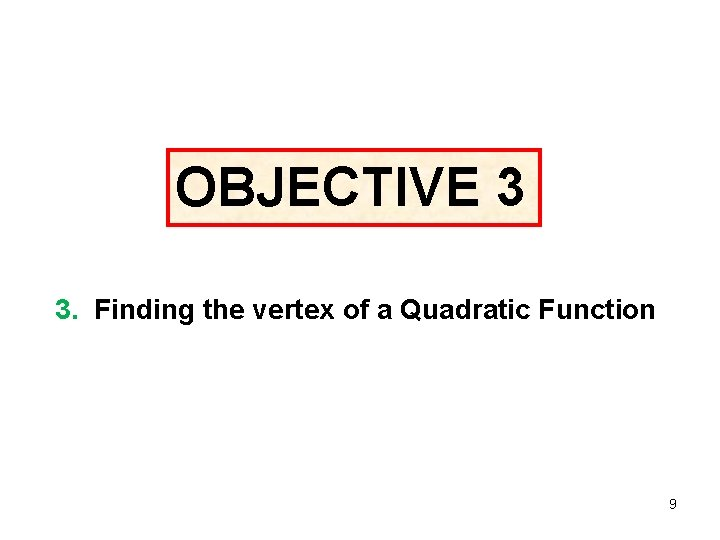OBJECTIVE 3 3. Finding the vertex of a Quadratic Function 9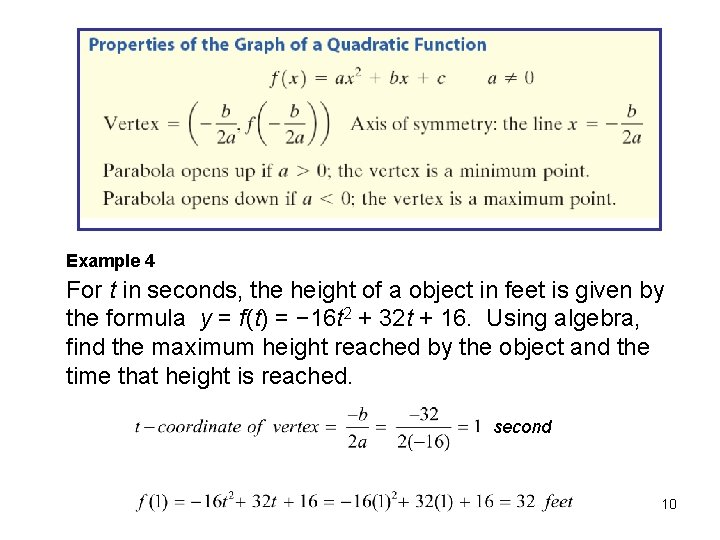Example 4 For t in seconds, the height of a object in feet is given by the formula y = f(t) = − 16 t 2 + 32 t + 16. Using algebra, find the maximum height reached by the object and the time that height is reached. second 10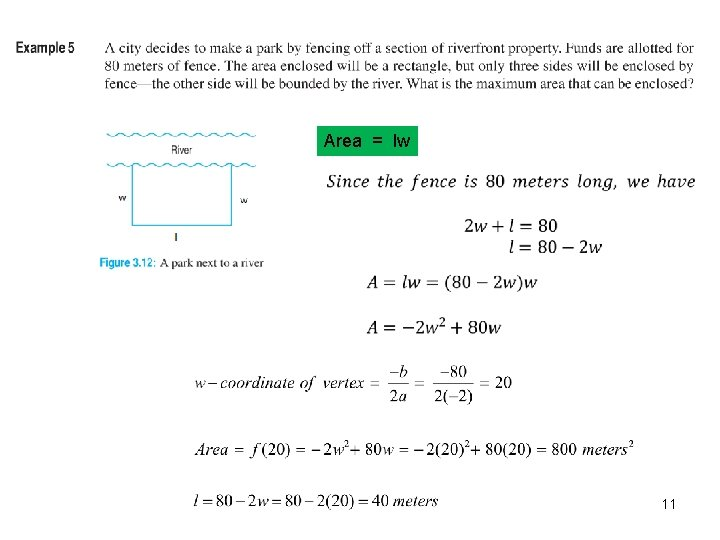Area = lw 11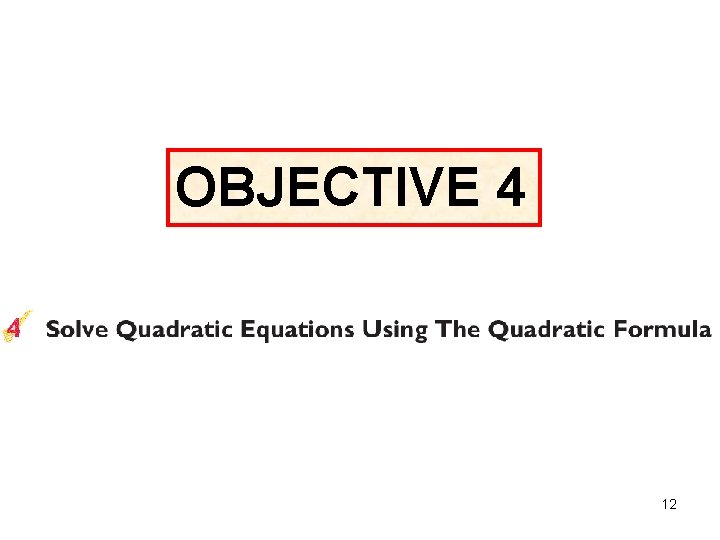OBJECTIVE 4 12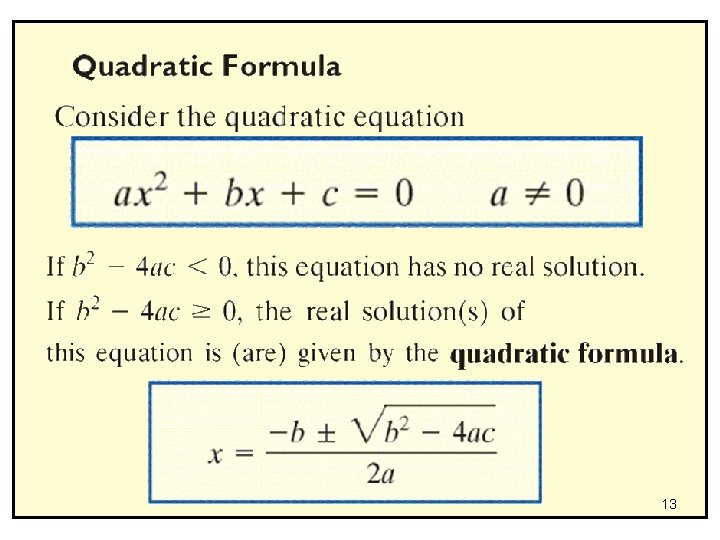13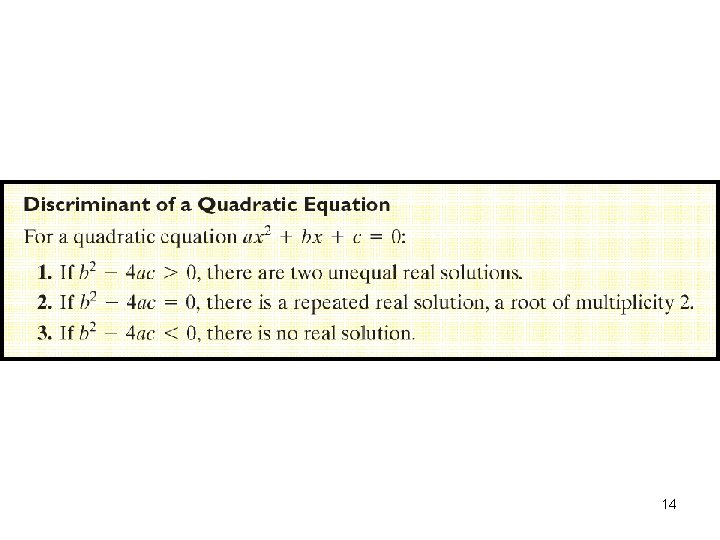14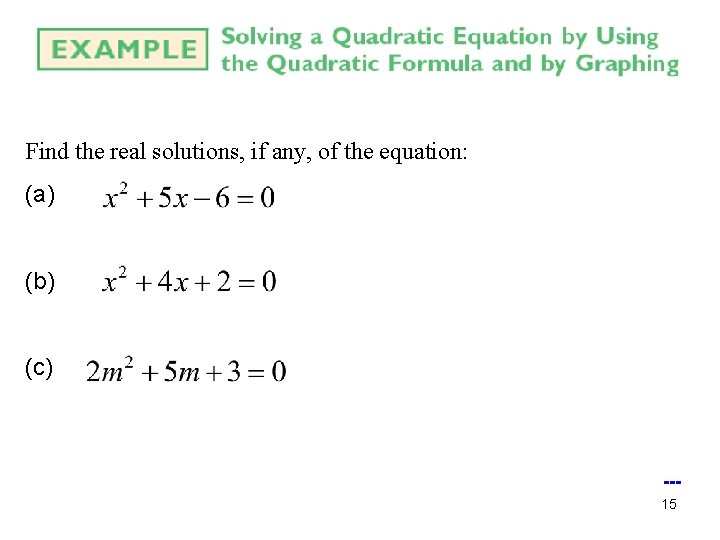Find the real solutions, if any, of the equation: (a) (b) (c) --15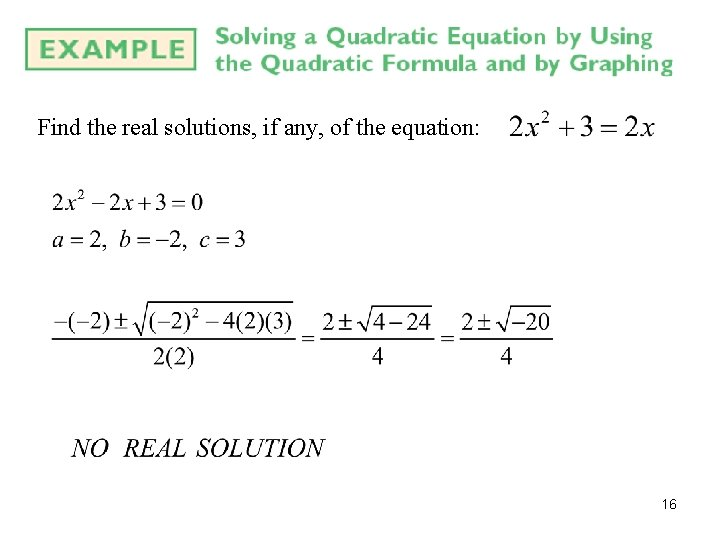Find the real solutions, if any, of the equation: 16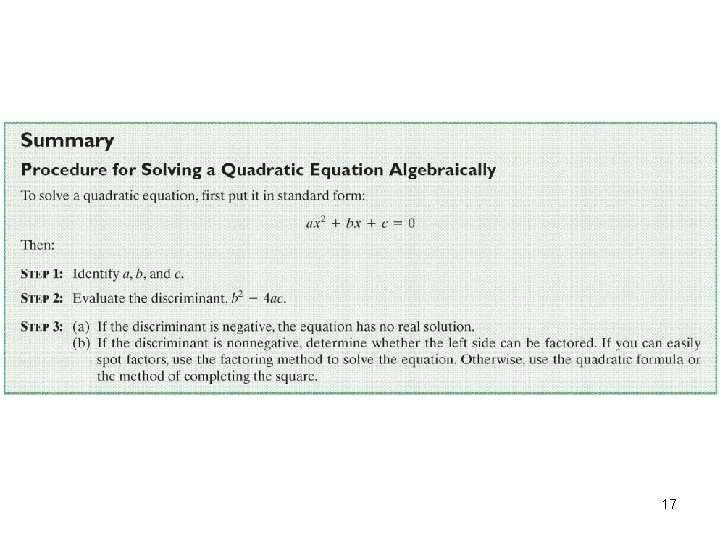17OBJECTIVE 5 18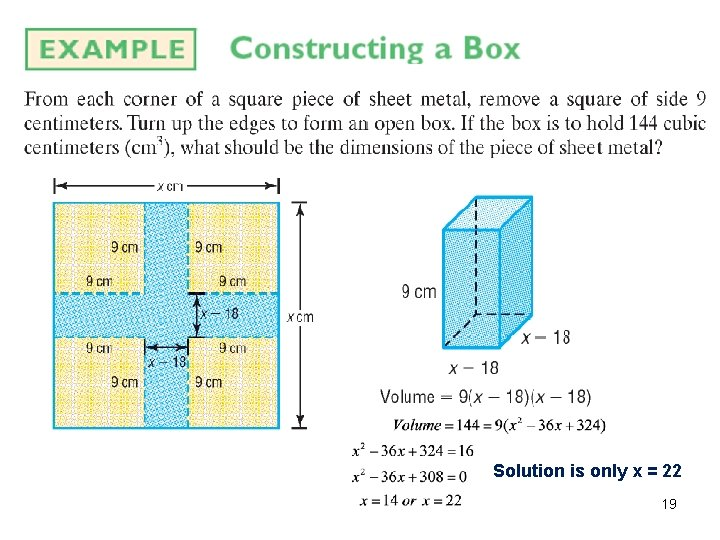Solution is only x = 22 19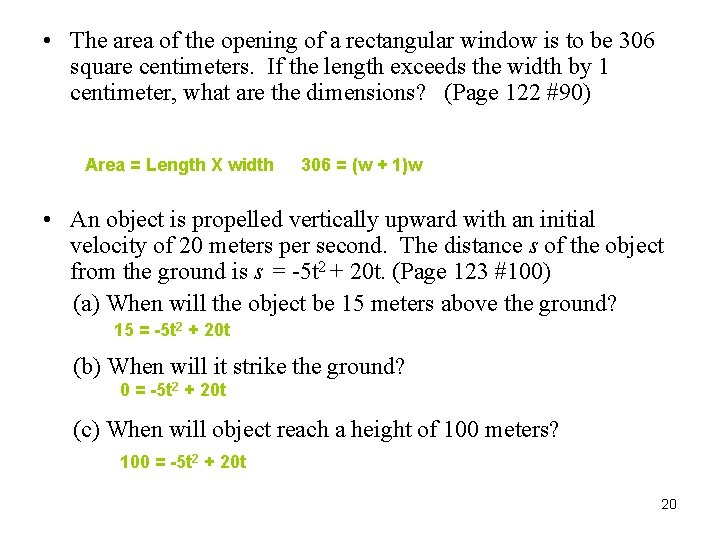• The area of the opening of a rectangular window is to be 306 square centimeters. If the length exceeds the width by 1 centimeter, what are the dimensions? (Page 122 #90) Area = Length X width 306 = (w + 1)w • An object is propelled vertically upward with an initial velocity of 20 meters per second. The distance s of the object from the ground is s = -5 t 2 + 20 t. (Page 123 #100) (a) When will the object be 15 meters above the ground? 15 = -5 t 2 + 20 t (b) When will it strike the ground? 0 = -5 t 2 + 20 t (c) When will object reach a height of 100 meters? 100 = -5 t 2 + 20 t 20